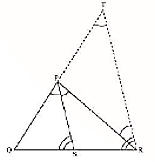Guru

# In Figure, PS is the bisector of ∠ QPR of ∆ PQR. Prove that QS/PQ = SR/PR Q.1

• 0

How i solve the problem of class 10th of triangles chapter of exercise 6.6 of ncert of question no 1. How i solve this question because i don’t know how to solve it In Figure, PS is the bisector of ∠ QPR of ∆ PQR. Prove that QS/PQ = SR/PR

Share

1. Let us draw a line segment RT parallel to SP which intersects extended line segment QP at point T.

Given, PS is the angle bisector of ∠QPR. Therefore,

∠QPS = ∠SPR………………………………..(i)As per the constructed figure,

∠SPR=∠PRT(Since, PS||TR)……………(ii)

∠QPS = ∠QRT(Since, PS||TR) …………..(iii)

From the above equations, we get,

∠PRT=∠QTR

Therefore,

PT=PR

In △QTR, by basic proportionality theorem,

QS/SR = QP/PT

Since, PT=TR

Therefore,

QS/SR = PQ/PR

Hence, proved.

• 0